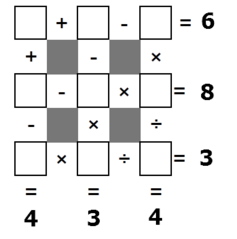# Can You Crack This Number GridUsing the digits 1 through 9 without repetition, fill out the number grid above. What is the product of the numbers in the 4 corners?

Note: The order in which this grid calculates is left-to-right/top-to-bottom unlike the usual order of operations. E.g., $1+2\times 3 = (1+2)\times3=9.$

×